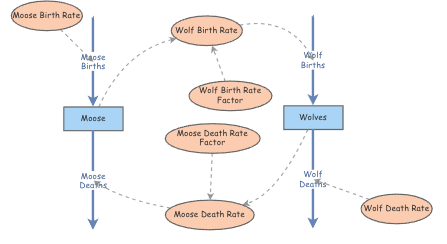# Stocks and Flows NotationAs I’ve learned about system dynamics I discovered the concept of stocks and flows. Stocks and Flows are useful for simulating the changes of a population over time and it occurred to me that you could create a very simple notation that was both readable by human and computers (e.g. configuring a simulation). This notation is an attempt to describe my approach.

A Stock and Flow diagram consists of one or more sequence chains which are iterated over as part of the execution of the simulation. The diagram itself requires only at least two arguments in specific order: start - The starting time unit for the simulation duration - The total number of time units to iterate time_unit - The timescale for the simulation (seconds, minutes, hours, days, weeks, months, years)

Any remaining arguments will be considered initial parameters to populate the system.

The vocabulary of the diagram is quite simple:

``````1
2
3
4
5
6
7
8
//                              // Comment
StockAndFlow(start, length, *)  // Start of the diagram with an optional arbitrary number of initial parameters to populate the system
=[ :some_stock ]=                 // Stock are levels of a quantity in the system
={ :symbol }=                   // Moves contents between Stocks, consists of at least one flow rate calculation
:"some text" or :some_text      // Variable
-->                             // Link shows a one-way directional relationship between two elements in the simulation.
+=                              // Start of the chain
=-                              // End of chain
``````
``````1
2
3
4
5
6
7
8
9
10
11
12
13
14
15
16
17
18
19
20
21
// Moose and Wolf System
StockAndFlow(start, duration, time_unit, *)

// Variables
:moose_birth_rate = 0.25
:wolf_birth_rate_factor = 0.000203
:wolf_birth_rate = wolf_birth_rate_factor * :moose
:moose_death_rate_factor = 0.0081
:moose_death_rate = moose_death_rate_factor * :wolves